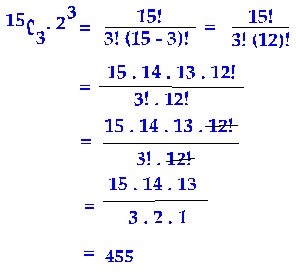## Binomial coefficient of any power of x

#### 7. Binomial Coefficient of any power of x in the binomial expansion:

Suppose we need to find the binomial coefficient of x27 in (x2+ 2x )15
Let us see the method step by step as follows:

1. First, we will have to find the term in which x27 occurs. To find a term implies finding r. So, we will now find r.

2. To find r, we will need the general term. Remember the general term? It is:3. In x27, since the power of x is 27, we should notice that in the general term having xn – r ,we should set 27 in n – r.But not so early. Why? Notice the next step 4

4. First collect all the powers of x in the given binomial expansion
(x2+2x )15
using the general term.

Now, let us apply all the four steps discussed above to find the binomial coefficient of x27in ( x2 + 2x )15. First, write the general term

#### Tr + 1 = ncr .xn – r . yr .………….. (A)

(You must compare the given binomial expansion ( x2 + 2x )15 with the standard form ( x + y )n in order to write x and y in the general term in (A) above).

From comparison, we see that x2 in the given form stands for x in the standard form, while 2x in the given form stands for y in the standard form. In short, x = x 2 and y = 2x Therefore, in the given binomial expansion (x2 + 2x )15 the general term is:

Tr + 1 = 15cr . (x2) n – r . (2x) r

Now,

= 15cr . (x 2 n – 2 r ) .( 2r xr )
= 15cr . (x 2 n – 2 r + r ) . ( 2r )
= 15cr . (x 2 n – r ) . ( 2r )………….. (B)

Since we need x27 ,we observe that we must set 27 in 2n – r in (B) above. 2n – r = 27.

Substitute n = 15,

2(15) – r = 27

r = 3.

Since r = 3, Tr + 1 = T 3 + 1 = T4

So, it is the fourth term that contains x 27

Apply r = 3 in (B) above, to write the binomial coefficient of x 27. It is